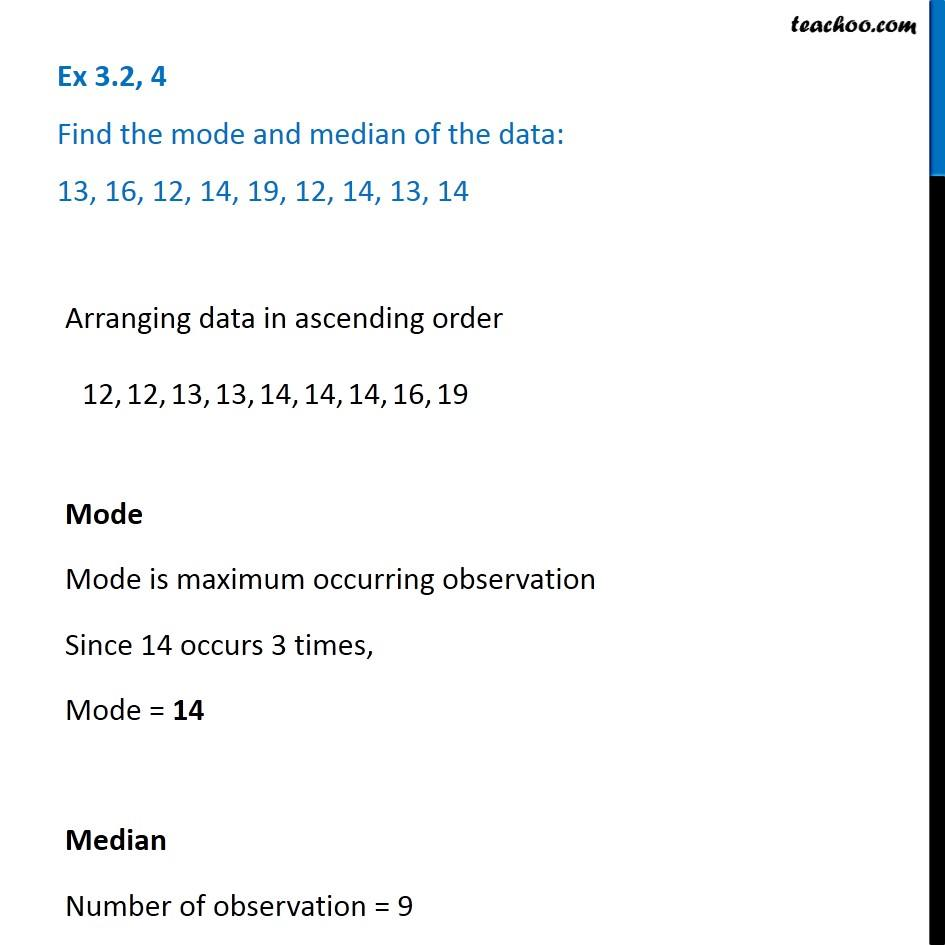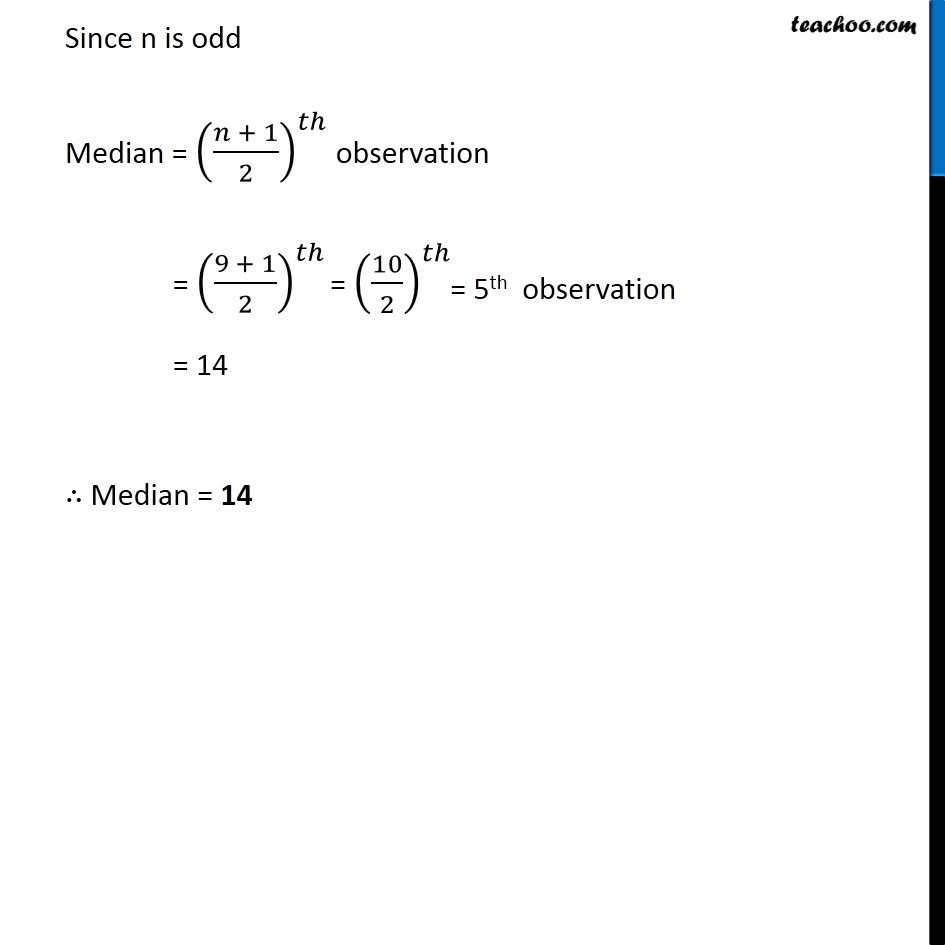Ex 3.2

Chapter 3 Class 7 Data Handling
Serial order wise### Transcript

Ex 3.2, 4 Find the mode and median of the data: 13, 16, 12, 14, 19, 12, 14, 13, 14 Arranging data in ascending order Mode Mode is maximum occurring observation Since 14 occurs 3 times, Mode = 14 Median Number of observation = 9 Since n is odd Median = ((𝑛 + 1)/2)^𝑡ℎ observation = ((9 + 1)/2)^𝑡ℎ = 14 ∴ Median = 14